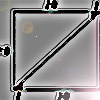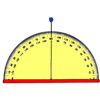# Resources tagged with: Sine, cosine, tangent

Filter by: Content type:
Age range:
Challenge level:

### There are 34 results

Broad Topics > Pythagoras and Trigonometry > Sine, cosine, tangent### Squ-areas

##### Age 14 to 16Challenge Level

Three squares are drawn on the sides of a triangle ABC. Their areas are respectively 18 000, 20 000 and 26 000 square centimetres. If the outer vertices of the squares are joined, three more. . . .### Inscribed in a Circle

##### Age 14 to 16Challenge Level

The area of a square inscribed in a circle with a unit radius is, satisfyingly, 2. What is the area of a regular hexagon inscribed in a circle with a unit radius?### Six Discs

##### Age 14 to 16Challenge Level

Six circular discs are packed in different-shaped boxes so that the discs touch their neighbours and the sides of the box. Can you put the boxes in order according to the areas of their bases?### From All Corners

##### Age 14 to 16Challenge Level

Straight lines are drawn from each corner of a square to the mid points of the opposite sides. Express the area of the octagon that is formed at the centre as a fraction of the area of the square.### Figure of Eight

##### Age 14 to 16Challenge Level

On a nine-point pegboard a band is stretched over 4 pegs in a "figure of 8" arrangement. How many different "figure of 8" arrangements can be made ?### Circle Box

##### Age 14 to 16Challenge Level

It is obvious that we can fit four circles of diameter 1 unit in a square of side 2 without overlapping. What is the smallest square into which we can fit 3 circles of diameter 1 unit?### Circle Scaling

##### Age 14 to 16Challenge Level

Describe how to construct three circles which have areas in the ratio 1:2:3.### Round and Round

##### Age 14 to 16Challenge Level

Prove that the shaded area of the semicircle is equal to the area of the inner circle.### Circumnavigation

##### Age 14 to 16Challenge Level

The sides of a triangle are 25, 39 and 40 units of length. Find the diameter of the circumscribed circle.### Farhan's Poor Square

##### Age 14 to 16Challenge Level

From the measurements and the clue given find the area of the square that is not covered by the triangle and the circle.### Where Is the Dot?

##### Age 14 to 16Challenge Level

A dot starts at the point (1,0) and turns anticlockwise. Can you estimate the height of the dot after it has turned through 45 degrees? Can you calculate its height?### At a Glance

##### Age 14 to 16Challenge Level

The area of a regular pentagon looks about twice as a big as the pentangle star drawn within it. Is it?### Cosines Rule

##### Age 14 to 16Challenge Level

Three points A, B and C lie in this order on a line, and P is any point in the plane. Use the Cosine Rule to prove the following statement.### Raising the Roof

##### Age 14 to 16Challenge Level

How far should the roof overhang to shade windows from the mid-day sun?### Screen Shot

##### Age 14 to 16Challenge Level

A moveable screen slides along a mirrored corridor towards a centrally placed light source. A ray of light from that source is directed towards a wall of the corridor, which it strikes at 45 degrees. . . .### A Scale for the Solar System

##### Age 14 to 16Challenge Level

The Earth is further from the Sun than Venus, but how much further? Twice as far? Ten times?### Lying and Cheating

##### Age 11 to 14Challenge Level

Follow the instructions and you can take a rectangle, cut it into 4 pieces, discard two small triangles, put together the remaining two pieces and end up with a rectangle the same size. Try it!### Eight Ratios

##### Age 14 to 16Challenge Level

Two perpendicular lines lie across each other and the end points are joined to form a quadrilateral. Eight ratios are defined, three are given but five need to be found.### Dodecawhat

##### Age 14 to 16Challenge Level

Follow instructions to fold sheets of A4 paper into pentagons and assemble them to form a dodecahedron. Calculate the error in the angle of the not perfectly regular pentagons you make.### Orbiting Billiard Balls

##### Age 14 to 16Challenge Level

What angle is needed for a ball to do a circuit of the billiard table and then pass through its original position?### Sine and Cosine for Connected Angles

##### Age 14 to 16Challenge Level

The length AM can be calculated using trigonometry in two different ways. Create this pair of equivalent calculations for different peg boards, notice a general result, and account for it.### Moving Squares

##### Age 14 to 16Challenge Level

How can you represent the curvature of a cylinder on a flat piece of paper?### The History of Trigonometry- Part 1

##### Age 11 to 18

The first of three articles on the History of Trigonometry. This takes us from the Egyptians to early work on trigonometry in China.### History of Trigonometry - Part 2

##### Age 11 to 18

The second of three articles on the History of Trigonometry.### History of Trigonometry - Part 3

##### Age 11 to 18

The third of three articles on the History of Trigonometry.### Making Maths: Clinometer

##### Age 11 to 14Challenge Level

You can use a clinometer to measure the height of tall things that you can't possibly reach to the top of, Make a clinometer and use it to help you estimate the heights of tall objects.### Doesn't Add Up

##### Age 14 to 16Challenge Level

In this problem we are faced with an apparently easy area problem, but it has gone horribly wrong! What happened?### Round and Round a Circle

##### Age 14 to 16Challenge Level

Can you explain what is happening and account for the values being displayed?### Far Horizon

##### Age 14 to 16Challenge Level

An observer is on top of a lighthouse. How far from the foot of the lighthouse is the horizon that the observer can see?### Sine and Cosine

##### Age 14 to 16Challenge Level

The sine of an angle is equal to the cosine of its complement. Can you explain why and does this rule extend beyond angles of 90 degrees?### Coke Machine

##### Age 14 to 16Challenge Level

The coke machine in college takes 50 pence pieces. It also takes a certain foreign coin of traditional design...### Muggles, Logo and Gradients

##### Age 11 to 18

Logo helps us to understand gradients of lines and why Muggles Magic is not magic but mathematics. See the problem Muggles magic.### Stadium Sightline

##### Age 14 to 18Challenge Level

How would you design the tiering of seats in a stadium so that all spectators have a good view?### 8 Methods for Three by One

##### Age 14 to 18Challenge Level

This problem in geometry has been solved in no less than EIGHT ways by a pair of students. How would you solve it? How many of their solutions can you follow? How are they the same or different?. . . .